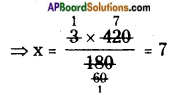# AP Board 6th Class Maths Solutions Chapter 6 Basic Arithmetic Ex 6.3

AP State Syllabus AP Board 6th Class Maths Solutions Chapter 6 Basic Arithmetic Ex 6.3 Textbook Questions and Answers.

## AP State Syllabus 6th Class Maths Solutions 6th Lesson Basic Arithmetic Ex 6.3

Question 1.
If the cost of 3 apples is Rs. 60/-, then find the cost of 7 apples.
Cost of 3 apples = Rs. 60
Cost of 1 apple = $$\frac{60}{3}$$ = Rs. 20
∴ Cost of 7 apples = 7 × Rs. 20 = Rs. 140

Question 2.
Uma bought 8 books for a total of ? 120. How much would she pay for just 5 books?
Cost of 8 books = Rs. 120
Cost of 1 book = $$\frac{120}{8}$$ = Rs. 15
∴ Cost of 5 books = 5 × 15 = Rs. 75Question 3.
The cost of 5 fans is Rs. 11,000/-. Find the number of funs that can be purchased for Rs. 4,400/-.
Cost of 5 fans = Rs. 11000
Cost of 1 fan = $$\frac{11000}{5}$$ = Rs. 2200
Number of fans can be purchased for Rs. 4400 = 4400 ÷ cost of 1 fan = $$\frac{4400}{2200}$$ = 2

Question 4.
A car is moving at a constant speed covers a distance of 180 km in 3 hours. Find the time taken by the car to cover a distance of 420 km at the same speed.
Distance covered = 180 km
Time taken = 3 Hrs.
Distance to be covered = 420 km
Time required = ? = x (say)
Since the car is moving at a constant speed, we have
180 : 3 is as to 420 : x
180 : 3 :: 420 : x
Its a proportion.
So, product of extremes = Product of means
180 × x = 3 × 420∴ Time required = 7 Hrs.
(OR)
Distance covered by a car in 3 hours = 180 km
Distance covered by a car in 1 hour = 180 ÷ 3
Time taken to cover 60 km distance = $$\frac{180}{3}$$ = 60 km
Time taken to cover 420 km distance = 420 ÷ 60 = $$\frac{420}{60}$$ = 7 hoursQuestion 5.
A truck requires 108 litres of diesel for covering a distance of 594 km. How much diesel will be required by the truck to cover a distance of 1650 km?
∴ x = $$\frac{108 \times 1650}{\hline 594}$$ = 300 litres
Distance covered by truck with 1 litre of diesel = $$\frac{594}{108}$$ km
Diesel required to cover 1650 km distance = 1650 ÷ $$\frac{594}{108}$$ = 1650 × $$\frac{108}{594}$$